# What is 3

What is the least common denominator of 11/15 and 12/19?

Result

d =  285

#### Solution:Leave us a comment of example and its solution (i.e. if it is still somewhat unclear...):

Showing 1 comment:#### To solve this example are needed these knowledge from mathematics:

Do you want to calculate least common multiple two or more numbers? Need help calculate sum, simplify or multiply fractions? Try our fraction calculator.

## Next similar examples:

1. Counting numberWhat is the smallest counting number divisible by 2,5,7,8 and 15?
2. Lcm of three numbersWhat is the Lcm of 120 15 and 5
3. FractionsThree-quarters of an unknown number are 4/5. What is 5/6 of this unknown number?
4. Reminder and quotientThere are given numbers A = 135, B = 315. Find the smallest natural number R greater than 1 so that the proportions R:A, R:B are with the remainder 1.
5. The florist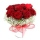The florist had 200 roses in the morning. During the day, more than half sold it. From the remaining roses, it will tie the bouquet. If a bouquet of 3, 4, 5 or 6 roses are bound, one always remains. How many roses from the morning shipment sold?
6. Class 9AOn the final certificate have one quarter of the class 9A mark "C" of mathematics, seventh mark "C" from the Czech language and two students failed in chemistry. How many students attend class 9A?
7. LCM of two numberFind the smallest multiple of 63 and 147
8. School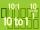Headteacher think whether the distribution of pupils in race in groups of 4,5,6,9 or 10. How many pupils must have at least school at possible options?
9. Dance ensemble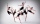The dance ensemble took the stage in pairs. During dancing, the dancers gradually formed groups of four, six and nine. How many dancers have an ensemble?
10. Trams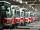Tram no. 3,7,10,11 rode together from the depot at 5am. Tram No. 3 returns after 2 hours, tram No. 7 an hour and half, no. 10 in 45 minutes and no. 11 in 30 minutes. For how many minutes and when these trams meet again?
11. Tailor master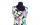There are less than 50m of textile in the tailoring workshop. When cutting on a blouse (consumption 1.5m), no textile is left. When using a cloth (consumption of 3.2m), no textile is left. How many meters of textile are in a tailor's workshop?
12. The port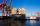Four ships Berthed in the port. Together sail from a port. The first ship will return to port every two weeks, second ship after 4 weeks, third after 8 weeks and fourth ship after 12 weeks. After how many weeks all the boats gather at the port?
13. Trams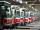Two trams started at the same time from the same place. One tram journey takes 30 minutes and the second 45 minutes to its final stop. How long will trams meet again?
14. Plum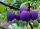On the platter are plum. How many were there if its have to be able to share equally among 8,10 and 12 children?
15. Simple equation 6Solve equation with one variable: X/2+X/3+X/4=X+4
16. Apples 14Maya bought 18 apples. She used 50% of the apples to make apple pies and 1/3 of the apples was mixed with other fruits to make salad. How many apples were left?
17. Sum of two primesChristian Goldbach, a mathematician, found out that every even number greater than 2 can be expressed as a sum of two prime numbers. Write or express 2018 as a sum of two prime numbers.